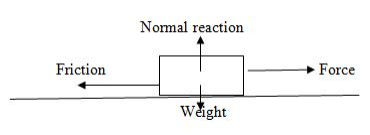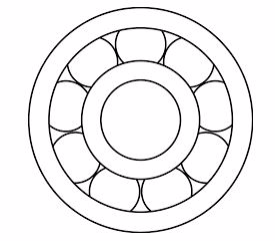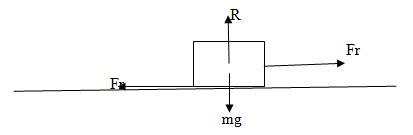Type Here to Get Search Results !

# PHYSICS FORM THREE TOPIC 2: FRICTIONFRICTION
The Concept of Friction
Explain the concept for friction
Friction is the force which opposes (resists) motion of the body for example: If a block of wood is placed on a table it remains at rest Unless it is acted upon by an external force.
The applied force must exceed the maximum friction force between the block of wood and the table if the block is to be moved along the table.Friction force between two surface in contact exists only when there is relative motion between the two contacting surface.
The friction between two surfaces exists because of the nature of the surface of the bodies in contact. (Strictly speaking all surfaces are rough because they are made up of peaks and valleys)
Demonstration
The peak and valleys on the surfaces of bodies may be due to a random arrangement of coarse particles, for example the surface of a gridding stones.
Smooth surfaces exert almost no friction. What happens when two objects are pushed?
When one body is pushed against another, the peaks of one surface have to rise up over the peaks of the other surface. Hence opposition to motion or friction occurs the force acting to restore the bodies to their original position.
Thefriction force has several advantages which includes the following:
1. It helps in walking process (because friction force stop us from slipping over).
2. It helps cars to move onroads easily due to friction between car tyres and road (makesa car stay on road) NOTE: Most car tyres have deep dreads to increase friction between the tyres and the road surface.
3. it helps in car braking system. Brakes rely on friction between the brake drum or pads and the wheels. In a bicycle, there are brake pads which clamp onto the wheel to slow it down.
Frictional force has several dis-advantages which includes the following:
1. Cause machinery to heat up and can cause wear and tear.
2. Cause machinery to be less efficient.
3. Cause machinery to produce noise.
Methods of Reducing Friction
Describe methods of reducing friction
Thedifferent methods ofreducing friction between surfaces includes the following:
1. Polishing:Polishing the surface, reduces the irregularities and hence, reduces friction.
2. Lubrication:It provides a layer of the lubricant on which the bodies can easily slide.
3. Use of ball-bearing or roller bearing:Roller bearing help to convert sliding friction into a milder form-rolling friction. Rolling friction is lesser than sliding friction.
4. Streamlining:Fast cars, boats, planes etc., have a streamlined body. This is to allow air (or water in the case of boats) to easily flow by, without offering much resistance. Flying birds have streamlined bodies.
5. Use of correct combination of surfaces in contact:Use of alloys on moving and sliding parts reduces friction because alloys have a low coefficient of friction.
Roller
• It is a simple cylinder on which a body to be pulled RESTS.
• It is used to eliminates sliding friction.
• Conveyor belts use metal rollers.Wheels
• These arerollers that are fixed to a moving body, held in place by cylinders or axles which are threaded in their centers. Example; a trailer towed by vehicle.
• Wheels are used to eliminate sliding friction.
• They are used to reduce friction (the friction between surfaces can be reduced by smoothing and polishing the surfaces in contact).
• In order to make surfaces slippery, a lubricant such as oil or graphite is used. Oil and Grease are commonly used invehicles and machines to reduce friction between moving parts. In engines, differential air is also an effective lubricant in machine.
Types of Friction
Identify types of friction
There are three main types of friction in daily life which include the following:
1. Static friction: an opposing forcebetween two solid objectsat rest.In simple words, when there is no relative motion between two solid objects in contact with each other, we describe the frictional force between them asstatic.
2. Limiting friction: numerically equal to the minimum external force required to make a body just move over another.
3. Dynamic friction: numerically equal to the force of opposition when a body is moving over the rough surface.
Limiting Friction
Determine limiting friction
Limiting friction is equal to the minimum external force required to make a body just move over one another. Is the maximum possible value of static friction. It is the frictional force that must be overcome before an object starts moving. The coefficient of friction will be the same for all masses. The limiting frictional forces is independent of applied force but depends on nature of surface.
Example 1
A block of mass 20 kg is pulled along a horizontal surface. If the coefficient of friction is 0.4, what force is acting on the block?
Solution
Force = coefficient of friction ×mass × acceleration due to gravity
F = 0.420×10 = 80N.

Laws of Friction
State laws of friction
The five laws of friction
1. When an object is moving, the friction is proportional and perpendicular to the normal force (N).
2. Friction is independent of the area of contact.
3. The coefficient of static friction is slightly greater than the coefficient of kinetic friction.
4. Within rather large limits, kinetic friction is independent of velocity.
5. Friction depends upon the nature of the surfaces in contact.
The Coefficient of Friction
Determine the coefficient of friction
Coefficient of friction is the ratio of the frictional force that acts between two objectsin contact to the normal reaction, R.Types of Coefficient of friction
There are three main types of coefficient of frictions which includes the following:
Coefficient of static friction is the ratio of the static friction to the normal reaction.
Us =Frs/R
Coefficient of dynamic friction US
Is the ratio of the dynamic friction to the normal reaction that acts onthe body.
Ud = Frd/R
Laws of Friction in Solving Problems
Apply laws of friction in solving problems
Demonstration to determine coefficient of dynamic friction.
Method of calculation
The coefficient of dynamic friction U is
U = Frictional force, Fr/Normal Reaction, R
U= Fr/R_______________________________ ( I)
By Resolving forces we get
Fr = W SinQ __________________________(II)
R= W CosQ ________________________(III)
Put Eqn (iii) ad (ii) into eqn (i)
U =W SinQ/Wc osQ
U =W SinQ/WCosQ
But SinQ/CosQ=TanQ
U=TanQ
Thus
TanQ = (AB/CB) FROM Angle ABC
But
U = TanQ
u = (ab/cb)
Example 2
Find the static friction between a block of wood of mass 10kg and the table on which it rests. A minimum force of 50N is required to make the block just move on the table top.Solution
Limiting Friction, Fr = 50N
Normal Reaction, r =(10 X 10 ) = 100N
Coefficient of static friction; Us
Us = (Fr/ R)
Us = (50/100)
Us = 0.5
Therefore,coefficient of static friction, Us = 0.5
Example 3
A mass is placed on an Inclined plane such that it moves at a constant speed when tapped tightly. If the angle the plane makes with the horizontal is 30º. Find the coefficient of dynamic friction.The Coefficient of friction U =Fr/R
Fr = WSinQ/R = WCosQ
At Equilibrium
U =WSinQ/wCosQ
U = (W/W) (SinQ/CosQ)
But (SinQ/CosQ) = TanQ
U = Tan 30º
U = 0.56
Coefficient of friction, U = 0.56

Tags

1.